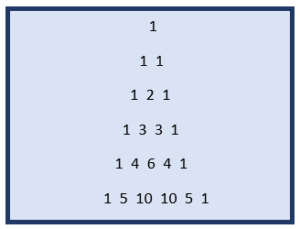# Everything you need to know About Pascal Triangle in JavaCreating Mathematical Patterns in any programming language is one of the foremost concepts. There is nothing different in the case of Java. In this article, we will understand how to implement different types of Pascal Triangle in Java.

## What is a Pascal Triangle?

A Pascal Triangle consists of binomial coefficients stored in a triangular array. It is one of the classic and basic examples taught in any programming language. We take an input n from the user and print n lines of the pascal triangle.

Example Of a Pascal TriangleA pascal triangle is started by first placing the number one in the first row and two ones in the second row. The later rows are bored by keeping the end elements same but the middle element is the sum of end elements from either side.

Consider the fourth row. The two middle terms are formed by adding one and two on either side and we get two three’s in between the two ones.

In other words, each number is directly added to others.

## Different Forms of Pascal Triangle in Java

CONSIDER THE CODE

Method 1:

```import java.util.Scanner;
public class PascalTri {
static void printTriangle(int n)
{

for (int line = 0; line < n; line++)
{
for (int i = 0; i <= line; i++)         System.out.print(findBinomial(line, i)+" ");                                   System.out.println();     }     }           static int findBinomial(int n, int j)     {         int result = 1;                   if (j > n - j)
j = n - j;

for (int i = 0; i < j; ++i)
{
result *= (n - i);
result /= (i + 1);
}
return result;
}

public static void main(String args[])
{
int n;
Scanner scn= new Scanner(System.in);
System.out.println("enter the number of rows");
n=scn.nextInt();
printTriangle(n);
}
}```

OUTPUT:

##EXPLANATION

In this code, we try to print a pascal triangle in java. Two methods are declared, one method to print the triangle and the second method to find the binomial coefficients.

```static void printTriangle(int n)
{

for (int line = 0; line < n; line++)
{
for (int i = 0; i <= line; i++)
System.out.print(findBinomial(line, i)+" ");

System.out.println();
}
}```

We have printTriangle function which is used to print the triangle. Two for loops are present. One for loop takes care of the spaces and the second one takes care of the printing. The findBinomial function is called to find the terms.

findBinomial is used to find all the terms in the pascal triangle. We have one for loop to perform the calculations.

This method has order O(n^3) time complexity.

Method 2:

```import java.io.*;
import java.util.Scanner;
public class PascalTri {

public static void printPascal(int n)
{
for(int line = 1; line <= n; line++)
{

int C=1;
for(int i = 1; i <= line; i++)
{

System.out.print(C+" ");
C = C * (line - i) / i;
}
System.out.println();
}
public static void main (String[] args) {
int n = 5;
printPascal(n);
}
}```

OUTPUT:This code runs with time complexity of O(n^2). It is similar to the first method but is much faster. The same process is repeated.

With this, we come to an end of this Pascal Triangle in Java article. I hope you got an idea of what is a Pascal Triangle and the different ways to Implement a Pascal Triangle in Java.

Check out the Java training by Edureka, a trusted online learning company with a network of more than 250,000 satisfied learners spread across the globe. Edureka’s Java J2EE and SOA training and certification course is designed for students and professionals who want to be a Java Developer. The course is designed to give you a head start into Java programming and train you for both core and advanced Java concepts along with various Java frameworks like Hibernate & Spring.

Got a question for us? Please mention it in the comments section of this “Pascal Triangle in Java” blog and we will get back to you as soon as possible.

Upcoming Batches For Java Certification Training Course
Course NameDate
Java Certification Training Course

Class Starts on 7th October,2023

7th October

SAT&SUN (Weekend Batch)
View Details
Java Certification Training Course

Class Starts on 25th November,2023

25th November

SAT&SUN (Weekend Batch)
View DetailsREGISTER FOR FREE WEBINARThank you for registering Join Edureka Meetup community for 100+ Free Webinars each month